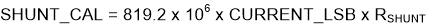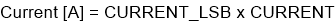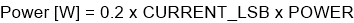SLYS026A June   2020  – June 2021

PRODUCTION DATA

1. Features
2. Applications
3. Description
4. Revision History
5. Pin Configuration and Functions
6. Specifications
7. Detailed Description
1. 7.1 Overview
2. 7.2 Functional Block Diagram
3. 7.3 Feature Description
4. 7.4 Device Functional Modes
5. 7.5 Programming
1. 7.5.1 I2C Serial Interface
6. 7.6 Register Maps
8. Application and Implementation
1. 8.1 Application Information
2. 8.2 Typical Application
9. Power Supply Recommendations
10. 10Layout
11. 11Device and Documentation Support
12. 12Mechanical, Packaging, and Orderable Information

• DGS|10

### 8.1.2 Current and Power Calculations

For the INA238-Q1 device to report current values in Ampere units, a constant conversion value must be written in the SHUNT_CAL register that is dependent on the maximum measured current and the shunt resistance used in the application. The SHUNT_CAL register is calculated based on Equation 1. The term CURRENT_LSB is the LSB step size for the CURRENT register where the current in Amperes is stored. The value of CURRENT_LSB is based on the maximum expected current as shown in Equation 2, and it directly defines the resolution of the CURRENT register. While the smallest CURRENT_LSB value yields highest resolution, it is common to select a higher round-number (no higher than 8x) value for the CURRENT_LSB in order to simplify the conversion of the CURRENT.

The RSHUNT term is the resistance value of the external shunt used to develop the differential voltage across the IN+ and IN– pins. Use Equation 1 for ADCRANGE = 0. For ADCRANGE = 1, the value of SHUNT_CAL must be multiplied by 4.

Equation 1.where

• 819.2 x 106 is an internal fixed value used to ensure scaling is maintained properly.
• the value of SHUNT_CAL must be multiplied by 4 for ADCRANGE = 1.

Equation 2.Note that the current is calculated following a shunt voltage measurement based on the value set in the SHUNT_CAL register. If the value loaded into the SHUNT_CAL register is zero, the current value reported through the CURRENT register is also zero.

After programming the SHUNT_CAL register with the calculated value, the measured current in Amperes can be read from the CURRENT register. The final value is scaled by CURRENT_LSB and calculated in Equation 3:

Equation 3.where

• CURRENT is the value read from the CURRENT register

The power value can be read from the POWER register as a 24-bit value and converted to Watts by using Equation 4:

Equation 4.where

• POWER is the value read from the POWER register.
• CURRENT_LSB is the lsb size of the current calculation as defined by Equation 2.

For a design example using these equations refer to Section 8.2.2.